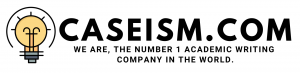# Portfolio Optimization Case Solution & Answer

## Portfolio Optimization Case Solution

On the other side, the total generated portfolio means shows less returns as compared to the previous scenario. Therefore it is concluded that the model shows less positive results for the particular investors regarding the holding of the stocks.

Efficiency frontier

Under the case, the results are similar to the previous scenario because the portfolio mean is directly proportional to the level of price changes overtime. So it is identified that both models shows the same results of changes but generate different outcomes due to the particular factors involved under each case.

Fama/French Five factors model

The net results under Fama/French Five factors model show relatively the same as in the case of three factors model, so it shows that sudden changes in the mean of prices and return would not change the efficiency frontier curve due to directly proportional with the volatility of prices. It has also determine that if the portion of prices from the historical perspective would calculate than the average deduction would involve due to less standard deviation involved in the previous years of stock under less price level.

Net portfolio

After analysing the all the scenarios of portfolio optimization, it is identified that under the best case, the traditional portfolio method to calculate the results would be preferable to the particular investors due to high risk and return factor involved in each industry. On the other side, Fama factor 3 period generated near and less than 1 which shows that the investors would not hold the shares for the future consideration.

The results under Fama factor 5 period in the first and second scenario shows that a moderate level of return would be generated due to the nature of a rate near to the risk-free rate. So after all, it is concluded that the most favourable industry for holding the particular stocks would be BusEq because it generates more returns under each of the given cases with moderate level of standard deviation involved in it.

Sharpe Ratio

With certain analysis of determining the Sharpe ratio of each of the given scenarios, it is concluded that the best option for the investors would be to go for the traditional portfolio method instead of applying to the other methods because it would generate positive Sharpe ratio, which represents more return as compared to the level of standard deviation and the other risk factors so investors should consider to hold the stock under the traditional way to optimize the given portfolio. …………………

This is just a sample partial work. Please place the order on the website to get your own originally done case solution